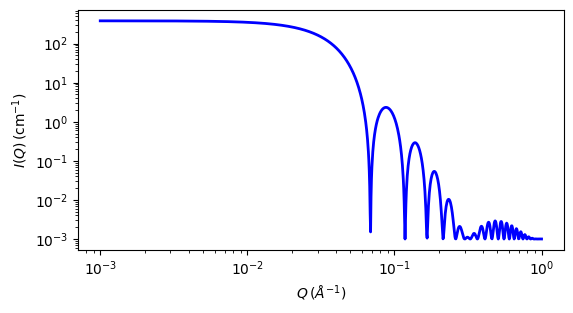# core_shell_sphere

Form factor for a monodisperse spherical particle with particle with a core-shell structure.

Parameter

Description

Units

Default value

scale

Scale factor or Volume fraction

None

1

background

Source background

cm-1

0.001

60

thickness

Sphere shell thickness

10

sld_core

core scattering length density

10-6-2

1

sld_shell

shell scattering length density

10-6-2

2

sld_solvent

Solvent scattering length density

10-6-2

3

The returned value is scaled to units of cm-1 sr-1, absolute scale.

This model provides the form factor, $$P(q)$$, for a spherical particle with a core-shell structure. The form factor is normalized by the particle volume.

For information about polarised and magnetic scattering, see the Polarisation/Magnetic Scattering documentation.

Definition

The 1D scattering intensity is calculated in the following way (Guinier, 1955)

$P(q) = \frac{\text{scale}}{V} F^2(q) + \text{background}$

where

$F(q) = \frac{3}{V_s}\left[ V_c(\rho_c-\rho_s)\frac{\sin(qr_c)-qr_c\cos(qr_c)}{(qr_c)^3} + V_s(\rho_s-\rho_\text{solv})\frac{\sin(qr_s)-qr_s\cos(qr_s)}{(qr_s)^3} \right]$

where $$V_s$$ is the volume of the whole particle, $$V_c$$ is the volume of the core, $$r_s$$ = $$radius$$ + $$thickness$$ is the radius of the particle, $$r_c$$ is the radius of the core, $$\rho_c$$ is the scattering length density of the core, $$\rho_s$$ is the scattering length density of the shell, $$\rho_\text{solv}$$, is the scattering length density of the solvent.

The 2D scattering intensity is the same as $$P(q)$$ above, regardless of the orientation of the $$q$$ vector.

NB: The outer most radius (ie, = radius + thickness) is used as the effective radius for $$S(Q)$$ when $$P(Q) \cdot S(Q)$$ is applied.

Validation

Validation of our code was done by comparing the output of the 1D model to the output of the software provided by NIST (Kline, 2006). Figure 1 shows a comparison of the output of our model and the output of the NIST software.Fig. 79 1D plot corresponding to the default parameters of the model.

Source

core_shell_sphere.py $$\ \star\$$ core_shell_sphere.c $$\ \star\$$ core_shell.c $$\ \star\$$ sas_3j1x_x.c

References

1. A Guinier and G Fournet, Small-Angle Scattering of X-Rays, John Wiley and Sons, New York, (1955)

Authorship and Verification

• Author: